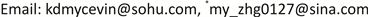1国家电网信息通信产业集团，北京中电普华信息技术有限公司，北京

2北京科技大学，自动化学院，北京1. 引言

2. 电力云资源池的基础架构

3. 电力云资源池投资成本监控算法3.1. 电力云资源池投资成本监控算法

Y ( x ) = T ( x ) − Z ( x ) (1)

3.2. 成本分析

1) 资源池投资成本分析

C h ( x ) = c min ( x ) × N (2)

C R ( x ) = N × ϕ × 100 , 000 (3)

Composition cost of the typical mini-delivery unit. Unit: ten thousand Yua

C i ( x ) = β × N (4)

Z ( x ) = C h ( x ) + C r ( x ) + C i ( x ) (5)

2) 同等能力传统架构建设的成本

C h t ( x ) = 3 × N × c s (6)

C r t ( x ) + C i t ( x ) = C r ( x ) + C i ( x ) (7)

3.3. 算法的实践与分析

Y ( x ) = 3 × N × c s − c min × N (8)

Financial rewards of different scale resource pool. Unit: ten thousand Yua

512036062985400−898
61444326957.66480−477.6
71685047617.27560−57.2
81925768276.88640363.2
92166488936.49720783.6
10240720959610,8001204
20480144016,19221,6005408
30720216022,78832,4009612
40960288029,38443,20013,816
501200360035,98054,00018,020
1002400720068,960108,00039,040

4. 结论及展望